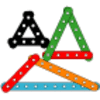#### You may also like### I'm Eight

Find a great variety of ways of asking questions which make 8.### Let's Investigate Triangles

Vincent and Tara are making triangles with the class construction set. They have a pile of strips of different lengths. How many different triangles can they make?### Noah

Noah saw 12 legs walk by into the Ark. How many creatures did he see?

# Are You Well Balanced?

##### Age 5 to 7 Challenge Level:

Try starting off by putting weights on the $1$ hook. How many will you need to balance with $10$?
What about trying the $2$ hook? How many will you need this time?
Can you keep going in this way?
If I had two weights on the $10$ hook, what would this be the same as in total?
If I then hang two weights on the other side, what would that balance with? So how many will I need on the $2$ hook to balance with the two on the $10$?